# GMAT Tricks with Systems of Equations: Part 1

A lot of GMAT test-takers vaguely remember a rule from high school, that it’s possible to solve for two variables if and only if you’re given two equations, and generally that it’s possible to solve for n variables if and only if you’re given n equations. Unfortunately, that rule isn’t quite correct as written, and even the correct rule isn’t always relevant.

Applying the rule incorrectly causes quite a few errors on the quant section, particularly with Data Sufficiency questions. This is the first in a series of posts on avoiding those errors:

## Let’s get the rule right!

Take a minute or two to answer this problem:

What is the value of ?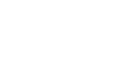(A) Statement (1) ALONE is sufficient, but statement (2) alone is not sufficient.
(B) Statement (2) ALONE is sufficient, but statement (1) alone is not sufficient.
(C) BOTH statements TOGETHER are sufficient, but NEITHER statement ALONE is sufficient.
(D) EACH statement ALONE is sufficient.
(E) Statements (1) and (2) TOGETHER are NOT sufficient.

If we too hastily apply the rule described above, we’ll probably choose (C) right away. After all, x  and y are two variables and (1) and (2) are two equations.  However, the correct answer is (E).  Notice that we can manipulate (1) to show that it is equivalent to (2):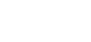Multiply through by 3.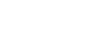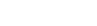That means that we have effectively just one equation. If you actually tried to solve for x and y just now, whether by substitution or by simultaneous solution, you probably ended up with something trivially true, like 0=0 or x=x. It turns out that the often-vaguely-remembered rule I cited above isn’t quite right. The rule isn’t actually that it’s possible to solve for two variables if and only if you’re given two equations.

## The correct rule for systems of equations

The correct rule is that a system with the same number of distinct linear equations and unknowns has a single unique solution. So if the equations aren’t distinct or aren’t linear, then the rule doesn’t apply.

Once in a great while a Data Sufficiency question will include two equivalent equations rather than two distinct equations. Here are four useful ways to think about equivalent equations (they all mean the same thing at bottom, so if even one of them makes sense to you that’s good enough):

• Equivalent equations describe the same line.
• Equivalent equations have the same solution sets.
• Equivalent equations can be transformed into one another.
• Equivalent equations are identical when expressed in slope-intercept form.

As I wrote though, the GMAT gives you equivalent equations only once in a great while. A typical 37-question quant section will have no such questions.

To be safe, once you’ve determined that neither (1) nor (2) is sufficient by itself and you’re left with answer choices (C) and (E), you can either attempt to transform one equation into the other or ascertain that the constants and coefficients in one equation aren’t all similar multiples of the constants and coefficients in the other. Notice that in the two equivalent equations above the ratios of the x terms (x  and 3x), the y terms (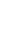and y), and the constants (3 and 9) are all the same, 1:3. If you can see that the equations you’re dealing with aren’t related in this way, then you should be fine.

One final note: If you arrive at two linear equations by translating a word problem into algebra, you can be sure that they’re distinct. I have never seen an exception to that rule, and there are a number of reasons that any exception would make a very poor GMAT question, so I don’t expect to see any exceptions. It’s not impossible, but it’s very unlikely.

## Author

•Michael Schwartz is really good at standardized tests. He’s earned multiple perfect scores on the GRE, GMAT, and LSAT. He’d rather have perfect pitch or be able to run low 1:40s for the 800 meters, but you take what you get. He has decades of teaching and curriculum-development experience. One of these days he might finish his dissertation and collect that Ph.D. in philosophy. Might.